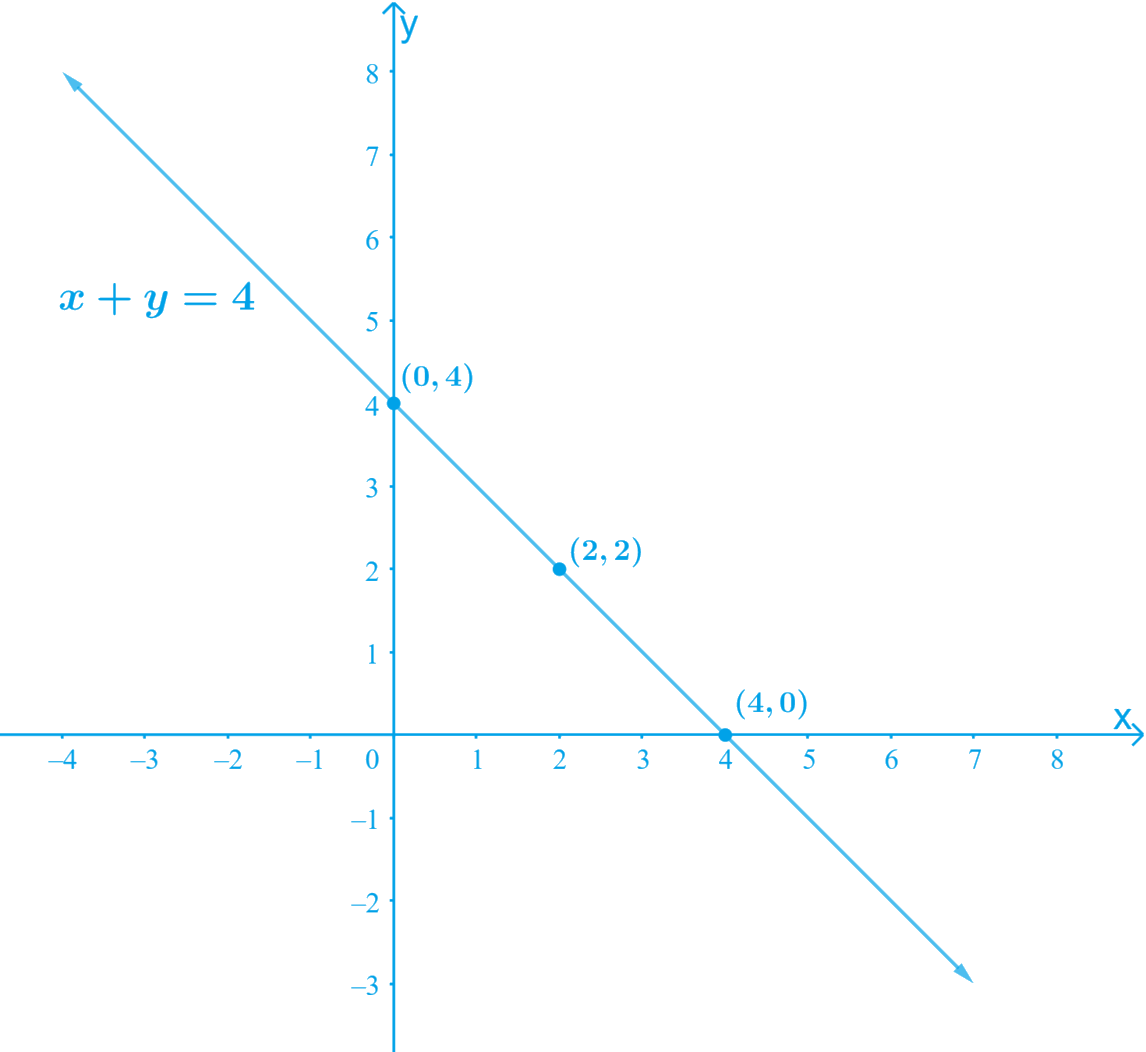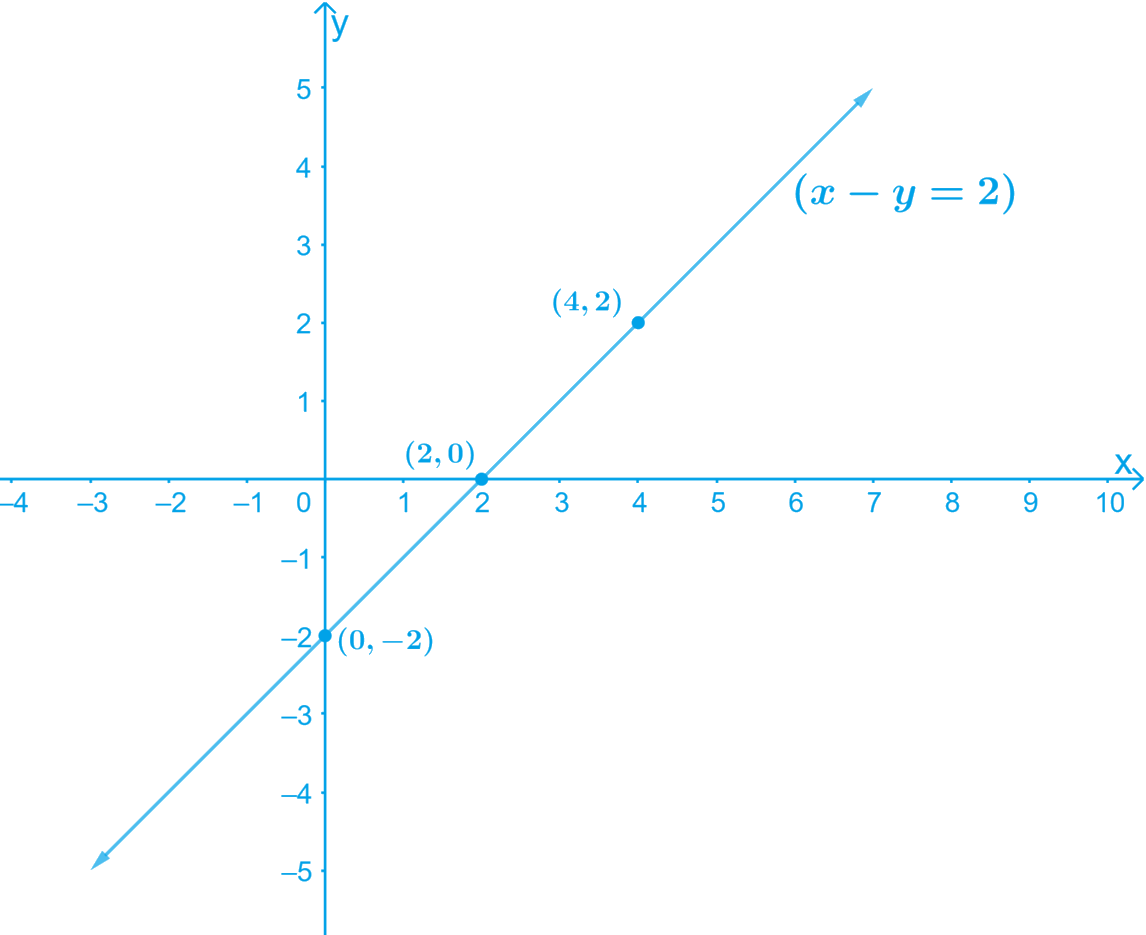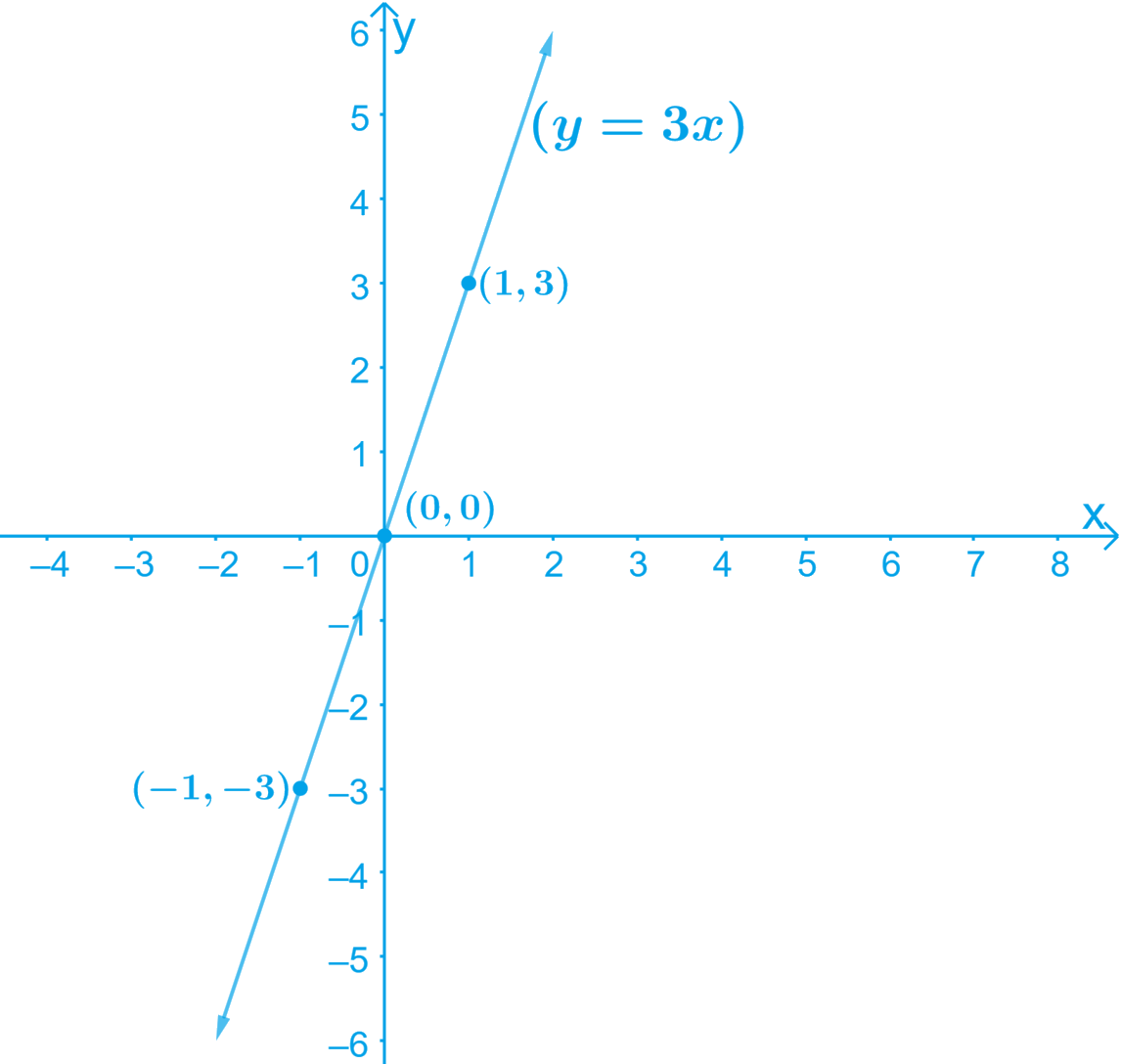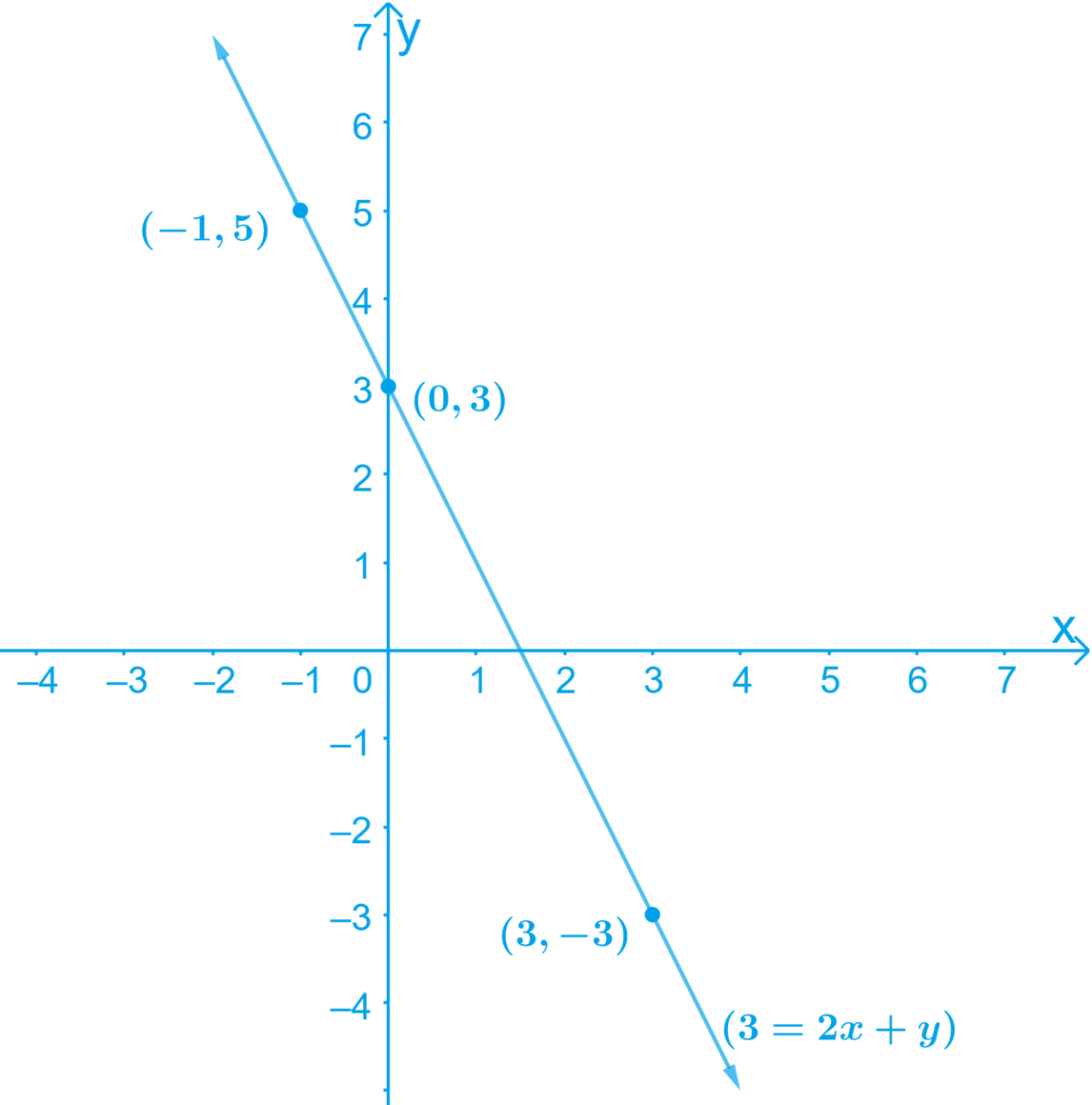# Ex.4.3 Q1 Linear Equations in Two Variables Solution - NCERT Maths Class 9

## Question

Draw the graph of each of the following linear equations in two variables:

(i) $$x + y = 4$$

(ii) $$x – y = 2$$

(iii) $$y = 3x$$

(iv) $$3 = 2x + y$$

Video Solution
Linear Equations In Two Variables
Ex 4.3 | Question 1

## Text Solution

What is known?

Linear equations

What is Unknown?

How to draw graph

Reasoning:

First of all, we can draw table for different values of $$x$$ and $$y$$ and then with the help of the values we can plot graph for each equation.

Steps:

(i) $$x + y = 4$$

Re-write the equation as

\begin{align}&x + y = 4 \\&\Rightarrow y = 4 \,– x \quad\dots\text{Equation } (1)\end{align}

By substituting the different values of $$x$$ in the Equation (1), we get different values for $$y$$

• When $$x = 0,$$ we have: $$y = 4 – 0 = 4$$
• When $$x = 2,$$ we have: $$y = 4 – 2 = 2$$
• When $$x = 4,$$ we have: $$y = 4 – 4 = 0$$

Thus, we have the following table with all the obtained solutions:

 $$x$$ $$0$$ $$2$$ $$4$$ $$y$$ $$4$$ $$2$$ $$0$$

By Plotting the points $$(0, 4) (2, 2)$$ and $$(4, 0)$$ on the graph paper and drawing a line joining the corresponding points, we obtain the Graph.

The graph of the line represented by the given equation is as shown.(ii) $$x – y = 2$$

Re-write the equation as
\begin{align}&x - y = 2\\&\Rightarrow y = x \,– 2\; \quad \dots\text{Equation } (1)\end{align}

By substituting the different values of $$x$$ in the Equation (1) we get different values for $$y$$

• When $$x = 0,$$ we have $$y = 0\, – 2 =\, – 2$$
• When $$x = 2,$$ we have $$y = 2 \,– 2 = 0$$
• When $$x = 4,$$ we have $$y = 4 \,– 2 = 2$$

Thus, we have the following table with all the obtained solutions:

 $$x$$ $$0$$ $$2$$ $$4$$ $$y$$ $$-2$$ $$0$$ $$2$$

By Plotting the points $$(0, –2)$$, (2, 0) and (4, 2) on the graph paper and drawing a line joining the corresponding points, we obtain the Graph.

The graph of the line represented by the given equation is as shown(iii) $$y = 3x$$

By substituting the different values of $$x$$ in the Equation (1) we get different values for $$y$$

• When $$x = 0,$$ we have: $$y = 3(0) = 0$$
• When $$x = 1,$$ we have: $$y = 3 (1) = 3$$
• When $$x =\,–1,$$ we have:

$$y = 3(–1) = \,– 3$$

Thus, we have the following table with all the obtained solutions:

 $$x$$ $$0$$ $$1$$ $$-1$$ $$y$$ $$0$$ $$3$$ $$-3$$

By Plotting the points $$(0, 0), (1, 3)$$ and

$$(–1, –3)$$ on the graph paper and drawing a line joining the corresponding points, we obtain the Graph.

The graph of the line represented by the given equation is as shown:(iv) $$3 = 2x + y$$

Re-write the equation as
\begin{align} y = 3 – 2x\; \quad \dots\text{Equation } (1)\end{align}

By substituting the different values of $$x$$ in the Equation (1) we get different values for $$y$$

• When $$x = 0,$$ we have:

$$y = 3 \,– 2(0) = 3 \,– 0 = 3$$

• When $$x = 3,$$ we have:

$$y = 3 \,– 2(3) = 3 \,– 6 = \,– 3$$

• When $$x = \,– 1,$$ we have:

$$y = 3 \,– 2 (–1) = 3 + 2 = 5$$

Thus, we have the following table with all the obtained solutions:

 $$x$$ 0 3 $$-1$$ $$y$$ 3 $$-3$$ 5

By Plotting the points $$(0, 3)$$, $$(3, –3)$$ and $$(–1, 5)$$ on the graph paper and drawing a line joining the corresponding points, we obtain the Graph.

The graph of the line represented by the given equation is as shown.Video Solution
Linear Equations In Two Variables
Ex 4.3 | Question 1
Learn from the best math teachers and top your exams

• Live one on one classroom and doubt clearing
• Practice worksheets in and after class for conceptual clarity
• Personalized curriculum to keep up with school